Markov Decision Process

By Prof. Seungchul Lee
http://iai.postech.ac.kr/
Industrial AI Lab at POSTECH

Source

• By David Silver's RL Course at UCL
• By Prof. Zico Kolter at CMU

# 1. Markov Decision Process¶

• So far, we analyzed the passive behavior of a Markov chain with rewards
• A Markov decision process (MDP) is a Markov reward process with decisions (or actions).

## 1.1. MDP Definition¶

Definition: A Markov Decision Process is a tuple $\langle S,\color{red}{A},P,R,\gamma \rangle$

• $S$ is a finite set of states
• $A$ is a finite set of actions
• $P$ is a state transition probability matrix
$$P_{ss'}^\color{red}{a} = P\left[S_{t+1} = s' \mid S_t = s, A_t = \color{red}{a} \right]$$
• $R$ is a reward function, $R_s^\color{red}{a} = \mathbb{E} \left[ R_{t+1} \mid S_t = s, A_t = \color{red}{a} \right] \quad \left(= \mathbb{E} \left[ R_{t+1} \mid S_t = s \right], \; \text{often assumed} \right)$
• $\gamma$ is a discount factor, $\gamma \in [0,1]$

Example: Startup MDP

• You run a startup company. In every state, you must choose between Saving money or Advertising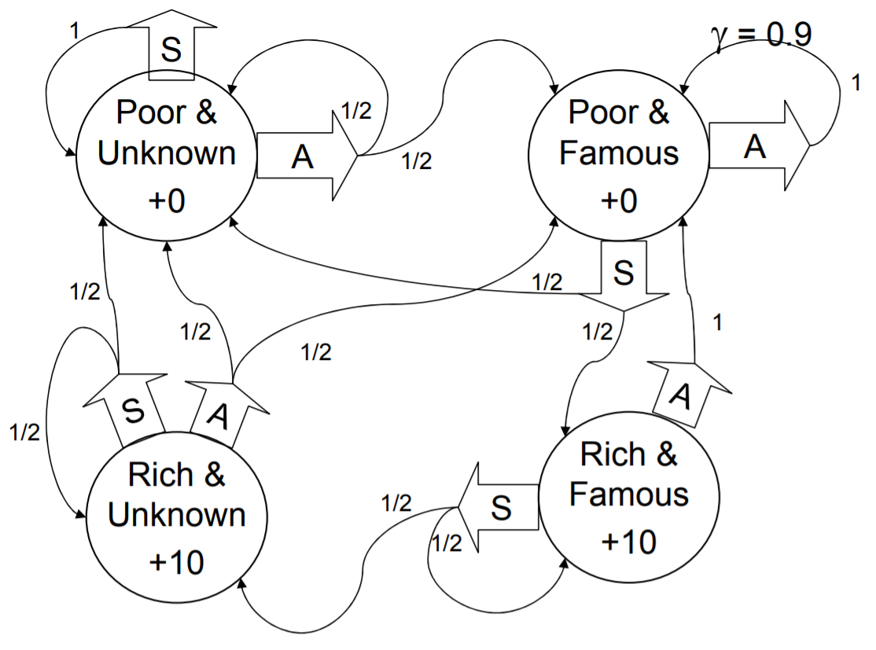## 1.2. Policies¶

• A policy is a mapping from state to actions, $\pi: S \rightarrow A$
• A policy fully defines the behavior of an agent
• It can be deteministic or stochastic
• Given a state, it specifies a distribution over actions
$$\pi (a \mid s) = P(A_t = a \mid S_t = s)$$
• MDP policies depend on the current state (not the history)
• Policies are stationary (time-independent, but it turns out to be optimal)
• Let $P^{\pi}$ be a matrix containing probabilities for each transition under policy $\pi$
• Given an MDP $\mathcal{M} = \langle S,A,P,R,\gamma \rangle$ and a policy $\pi$

• The state sequence $s_1, s_2, \cdots$ is a Markov process $\langle S, P^{\pi} \rangle$

• The state and reward sequence is a Markov reward process $\langle S,P^{\pi},R^{\pi},\gamma \rangle$

• Questions on MDP policy

• How many possible policies in our example?

• Which of the above two policies is best?

• How do you compute the optimal policy?

# 2. Bellman Equation¶

## 2.1. Value Function¶

State-value function

• The state-value function $v_{\pi}(s)$ of an MDP is the exptected return starting from state $s$, and then following policy $\pi$

\begin{align*} v_{\pi}(s) &= \mathbb{E}_{\pi} \left[G_t \mid S_t =s \right]\\ &= \mathbb{E}_{\pi}[ R_{t+1} + \gamma v_{\pi}(S_{t+1}) \mid S_t = s ] \end{align*}

Action-value function

• The action-value function $q_{\pi}(s,a)$ of an MDP is the exptected return starting from state $s$, taking action $a$, and then following policy $\pi$

\begin{align*} q_{\pi}(s,a) &= \mathbb{E}_{\pi} \left[G_t \mid S_t =s, A_t = a \right]\\ & = \mathbb{E}_{\pi} \left[R_{t+1} + \gamma q_{\pi} \left(S_{t+1}, A_{t+1} \right) \mid S_t =s, A_t = a \right] \end{align*}

## 2.2. Bellman Expectation Equation¶

### 2.2.1. Relationship between $v_{\pi}(s)$ and $q_{\pi}(s,a)$¶

• State-value function using policy $\pi$

$$v_{\pi}(s) = \sum_{a \in A}\pi(a \mid s) q_{\pi}(s,a)$$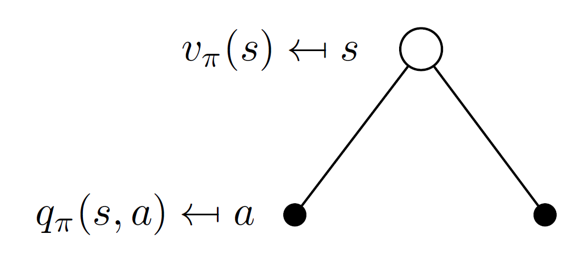• Action-value function using policy $\pi$

$$q_{\pi}(s,a) = R(s) + \gamma \sum_{s' \in S} P\left(s' \mid s,a \right) v_{\pi}\left(s'\right)$$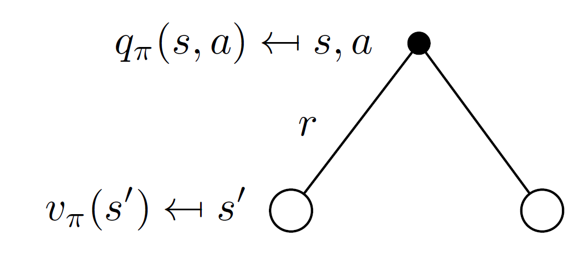### 2.2.2. Bellman Expectation Equation¶

• Bellman Expectation Equation for $v_{\pi}(s)$

\begin{align*} v_{\pi}(s) &= \sum_{a \in A}\pi(a \mid s) \underline{q_{\pi}(s,a)} \\ \\ &= \sum_{a \in A}\pi(a \mid s) \left( R(s) + \gamma \sum_{s' \in S} P\left(s' \mid s,a \right) v_{\pi}\left(s'\right) \right) \end{align*}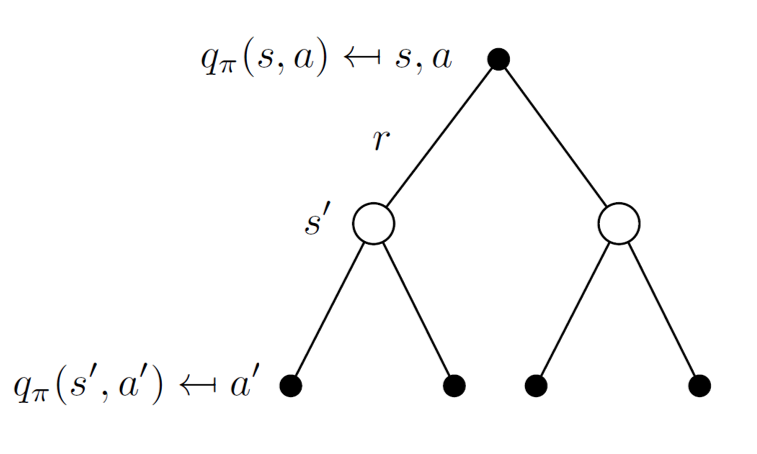• Bellman Expectation Equation for $q_{\pi}(s,a)$

\begin{align*} q_{\pi}(s,a) &= R(s) + \gamma \sum_{s' \in S} P\left(s' \mid s,a \right) \underline{v_{\pi} \left(s'\right)}\\ \\ &= R(s) + \gamma \sum_{s' \in S} P\left(s' \mid s,a \right) \left( \sum_{a' \in A} \pi \left(a' \mid s' \right) q_{\pi} \left(s', a' \right) \right) \end{align*}### 2.2.3. Solving the Bellman Expectation Equation¶

• The Bellman expectation equation can be expressed concisely in a matrix form,

$$v_{\pi} = R + \gamma \, P^{\pi} \,v_{\pi} \quad \implies \quad v_{\pi} = (I - \gamma \,P^{\pi})^{-1}R$$

• Iterative

$$v_{\pi} (s) \; \leftarrow \; R(s) + \gamma \sum_{s' \in S} P\left(s' \mid s, a \right) \;v_{\pi} \left(s' \right)$$

Example

• Given the policy 1, it is MRP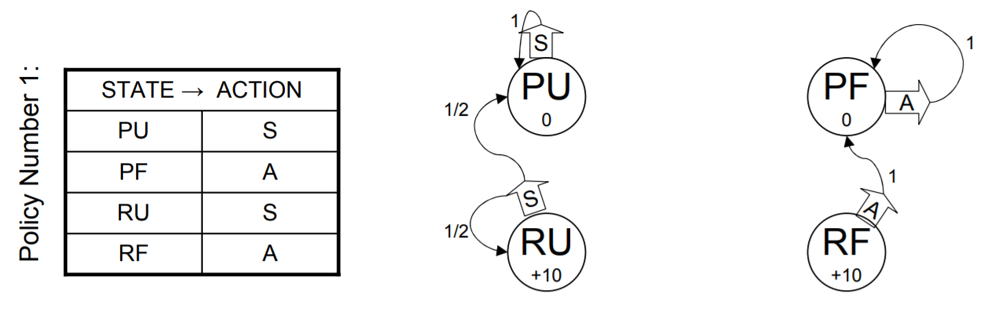$$v_{\pi} = R + \gamma \, P^{\pi} \,v_{\pi} \quad \implies \quad v_{\pi} = (I - \gamma \,P^{\pi})^{-1}R$$
In :
# [PU PF RU RF] = [0 1 2 3]

import numpy as np

P = [[1, 0, 0, 0],
[0, 1, 0, 0],
[0.5, 0, 0.5, 0],
[0, 1, 0, 0]]

R = [0, 0, 10, 10]

P = np.asmatrix(P)
R = np.asmatrix(R)
R = R.T

gamma = 0.9
v = (np.eye(4) - gamma*P).I*R

print(v)

[[ 0.        ]
[ 0.        ]
[18.18181818]
[10.        ]]

$$v_{\pi} (s) \; \leftarrow \; R(s) + \gamma \sum_{s' \in S} P\left(s' \mid s, a \right) \;v_{\pi} \left(s' \right)$$
In :
v = np.zeros([4,1])

for _ in range(10):
v = R + gamma*P*v

print(v)

[[ 0.        ]
[ 0.        ]
[18.17562716]
[10.        ]]

• Given the policy 2, it is MRP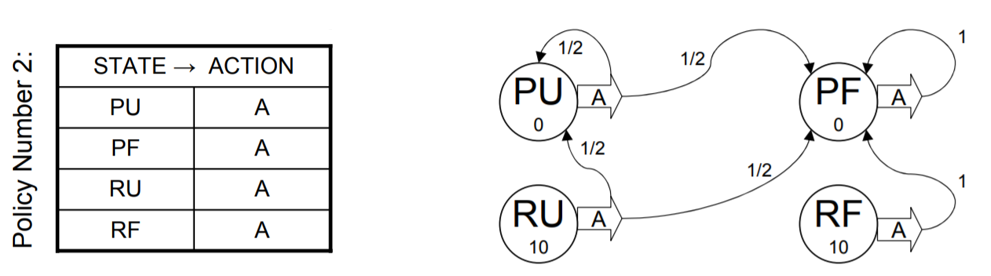In :
# [PU PF RU RF] = [0 1 2 3]

import numpy as np

P = [[0.5, 0.5, 0, 0],
[0, 1, 0, 0],
[0.5, 0.5, 0, 0],
[0, 1, 0, 0]]

R = [0, 0, 10, 10]

P = np.asmatrix(P)
R = np.asmatrix(R)
R = R.T

gamma = 0.9
v = (np.eye(4) - gamma*P).I*R
print(v)

[[ 0.]
[ 0.]
[10.]
[10.]]

In :
v = np.zeros([4,1])

for i in range(100):
v = R + gamma*P*v

print(v)

[[ 0.]
[ 0.]
[10.]
[10.]]


## 2.3. Bellman Optimality Equation¶

### 2.3.1. Optimal Value Function¶

• The optimal state-value functin $v_*(s)$ is the maximum value function over all policies

\begin{align*} v_*(s) &= \max_{\pi} v_{\pi}(s) \\ & = \max_a q_{\pi}(s,a) \\ & = \max_a \left( R(s) + \gamma \sum_{s' \in S} P(s' \mid s,a) v_{\pi}(s') \right)\\ & = R(s) + \gamma \max_a \sum_{s' \in S} P(s' \mid s,a) v_{\pi}(s') \end{align*}

• The optimal action-value functin $q_*(s,a)$ is the maximum action-value function over all policies

\begin{align*} q_*(s,a) &= \max_{\pi} q_{\pi}(s,a) \\ & = \max_{\pi} \left( R(s) + \gamma \sum_{s' \in S} P(s' \mid s,a) v_{\pi}(s') \right) \\ & = R(s) + \gamma \sum_{s' \in S} P(s' \mid s,a) \max_{\pi} v_{\pi}(s') \end{align*}

### 2.3.2. Optimal Policy¶

• The optimal policy is the policy that achieves the highest value for every state

$$\pi_{*}(s) = \arg\max_{\pi} v_{\pi}(s)$$

$\quad \;\;$and its optimal value function is written $v_{*}(s)$

• An optimal policy can be found by maximizing over $q_{*} (s,a)$

$$\pi_{*} (a \mid s) = \begin{cases} 1 \quad \text{if } a = \arg \max_{a \in A} q_{*}(s,a)\\ 0 \quad \text{otherwise} \end{cases}$$

• There is always a deterministic optimal policy for any MDP
• If we know $\pi_{*} (a \mid s)$, we immediately have the opimal policy

## 2.4. Solving the Bellman Optimality Equation¶

We can directly define the optimal value function using Bellman optimality equation

$$v_* (s) = R(s) + \gamma \max_{a} \sum_{s' \in S} P(s' \mid s, a) \;v_* \left(s' \right)$$

and optimal policy is simply the action that attains this max

$$\pi_*(s) = \arg\max_{a} \sum_{s' \in S} P\left(s' \mid s,a \right) \, v_* \left(s'\right)$$

• Bellman Optimality Equation is non-linear
• No closed form solution (in general)
• (Will learn later) many iterative solution methods
• Value Iteration
• Policy Iteration
• Q-learning
• SARSA
• You will get into details in the course of reinforcement learning

### 2.4.1. Value Iteration¶

Algorithm

$\quad$ 1. Initialize an estimate for the value function arbitrarily (or zeros)

$$v(s) \; \leftarrow \; 0 \quad \forall s \in S$$

$\quad$ 2. Repeat, update

$$v (s) \; \leftarrow \; R(s) + \gamma \max_{a} \sum_{s' \in S} P(s' \mid s,a) \;v \left(s' \right), \quad \forall s \in S$$

Note

• If we know the solution to subproblems $v_* \left(s' \right)$
• Then solution $v_* \left(s' \right)$ can be found by one-step lookahead

$$v (s) \; \leftarrow \; R(s) + \gamma \max_{a} \sum_{s' \in S} P\left(s' \mid s,a\right) \;v \left(s' \right), \quad \forall s \in S$$

• The idea of value iteration is to apply these updates iteratively

### 2.4.2. Policy Iteration¶

Algorithm

$\quad$ 1. initialize policy $\hat{\pi}$ (e.g., randomly)

$\quad$ 2. Compute a value function of policy, $v_{\pi}$ (e.g., via solving linear system or Bellman expectation equation iteratively)

$\quad$ 3. Update $\pi$ to be greedy policy with respect to $v_{\pi}$

$$\pi(s) \leftarrow \arg\max_{a} \sum_{s' \in S}P \left(s' \mid s,a \right) v_{\pi}\left(s'\right)$$

$\quad$ 4. If policy $\pi$ changed in last iteration, return to step 2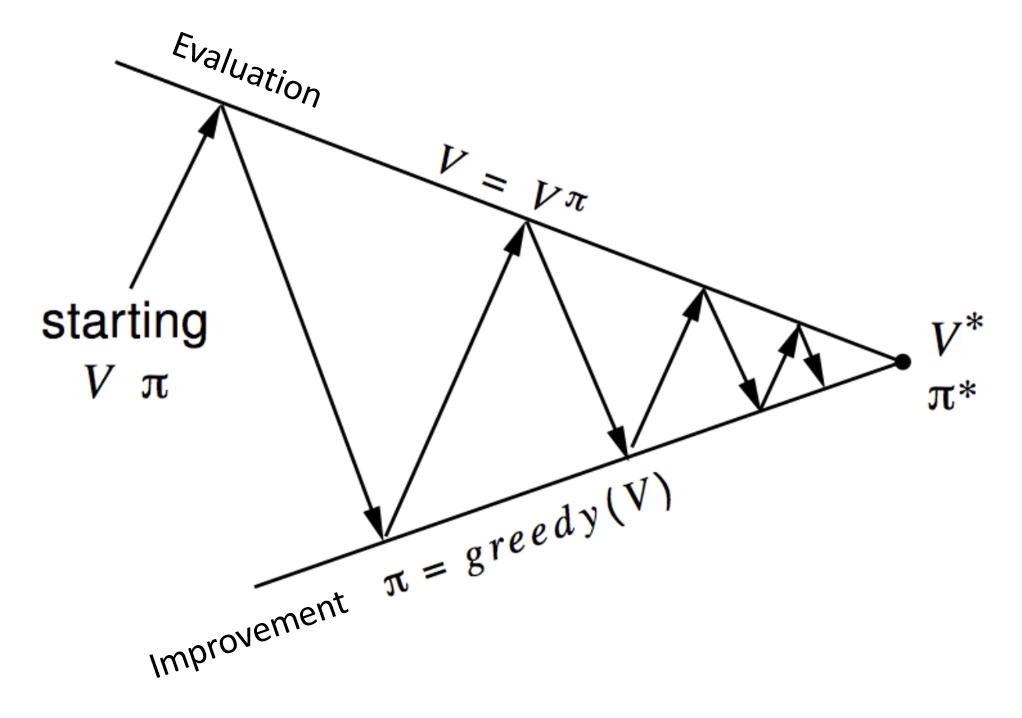Note

• Given a policy $\pi$, then evaluate the policy $\pi$

• Improve the policy by acting greedily with respect to $v_{\pi}$

• Policy iteration requires fewer iterations that value iteration, but each iteration requires solving a linear system instead of just applying Bellman operator

• In practice, policy iteration is often faster, especially if the transition probabilities are structured (e.g., sparse) to make solution of linear system efficient

In :
%%html

%%html